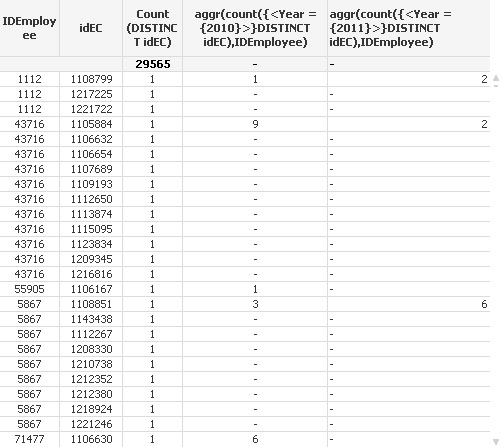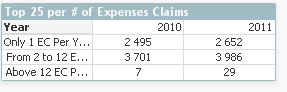# QlikView App Dev

Discussion Board for collaboration related to QlikView App Development.

Announcements
Join this live chat April 6, 10AM EST - QlikView to Qlik Sense REGISTER
cancel
Showing results for
Search instead for
Did you mean:Contributor II

## Sort out a straight table with frequency per year and Employee

Hello to all,

This is my first post and i thank you for any reply.

I am updating a QV base about expenses claims (EC) with 30 files each month.

In each file I have for each employee a various number of expenses claims with numerous type of expenses.

I would like to sort out a straight table with :

employee doing     only 1 EC per year

employee doing     from 2 to 12 EC per year

employee doing     more than 12 EC per year.

If i do a table with dimension =  'IDEmployee' and expression aggr(…) I will find an answer but this is not at all satisfactory for me.

I guess i should add an extracolumn during load ?

If you have any idear ?

Thank's again for any reply

Xavier

For example, with the screen shot below, i would like to have the following results :

Number of EC per Employee20102011
Only 1 EC per Year2
From 2 to 12 EC per Year33
Above 12 EC per year1 Solution

Accepted SolutionsMVP & Luminary

Hi,

Convert Straight table to Pivot table

Add year as Dimension.

Add the following following 3 expressions

Only 1 EC Per Year  =Sum(AGGR(If(Count(DISTINCT idEC) = 1, 1, 0), IDEmployee, Year))

From 2 to 12 EC Per Year =Sum(AGGR(If(Count(DISTINCT idEC) >= 2 AND Count(DISTINCT idEC) <= 12 , 1, 0),           IDEmployee, Year))

Above 12 EC Per Year - =Sum(AGGR(If(Count(DISTINCT idEC) > 12, 1, 0), IDEmployee, Year))

Finally pivot the expressions and years.Regards,

Jagan.

7 RepliesMVP & Luminary

Hi,

Can you attach a sample qvw file, so that it would be easier to work it out.

Regards,

jagan.Not applicable

Hi there,

It will be easier if you will use Pivot table.Contributor II
Author

Hi Jagan,

Hereto is a sample qvw file.

Hope it will be easier to understand.

Regards

XavierMVP & Luminary

Hi,

Convert Straight table to Pivot table

Add year as Dimension.

Add the following following 3 expressions

Only 1 EC Per Year  =Sum(AGGR(If(Count(DISTINCT idEC) = 1, 1, 0), IDEmployee, Year))

From 2 to 12 EC Per Year =Sum(AGGR(If(Count(DISTINCT idEC) >= 2 AND Count(DISTINCT idEC) <= 12 , 1, 0),           IDEmployee, Year))

Above 12 EC Per Year - =Sum(AGGR(If(Count(DISTINCT idEC) > 12, 1, 0), IDEmployee, Year))

Finally pivot the expressions and years.Regards,

Jagan.Contributor II
Author

Great Jagan,

It works perfectly.

Could you tell me how the aggr  function works? I am confused about it.

Regards

XavierMVP & Luminary

Aggr function calculates the Sum/Count by Fields regardless of Dimension.  Check the help file or online for further information.

Ex: AGGR(If(Count(DISTINCT idEC) = 1, 1, 0), IDEmployee, Year)

The above expression calculates the count by IDEmployee and Year.

It is similar to group by in SQL.

SELECT

Count(Distinct idEC)

FROM Table1

Group by IDEmployee, year;

Regards,

Jagan.Contributor II
Author

The help file is not so clear for a first approach.

Once again thanks for your help

regards

Xavier